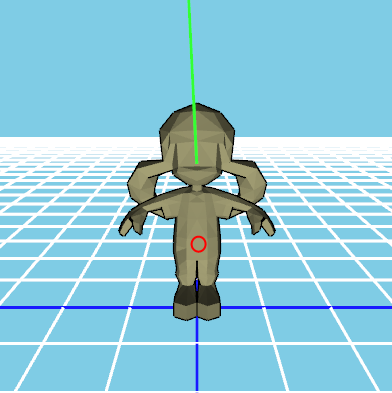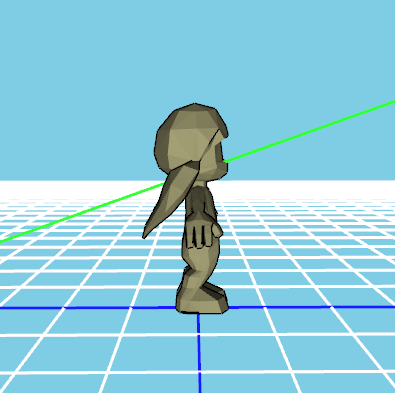# Ray triangle intersection problem.

I am calling gluUnProject(); with the mouse’s x coords and viewport- mouse’s y coord twice to get a starting and end point for my ray then subtracting the starting point from the end point and normalizing it to get the direction of the ray but the ray’s just going off where it wants.

The greenline is the ray and the red circle is where I clicked.As you can see the ray is very high up to where I clicked. It also moves along the x axis the furthur I click from the center.

When the ray intersects a triangle it’s ment to make the triangle go red. As you can see the triangle the ray did intersect didn’t go red. I have to get extremely close for the faces to go red but the ray never passes through the faces that are going red nor is the face that goes red under my mouse.

Here’s my code:

``````void Project(int x, int y)
{
GLdouble model_view;
GLdouble projection;
GLint viewport;
GLdouble NearX, NearY, NearZ, FarX, FarY, FarZ;
Vector Dir, Edge1, Edge2, H, S, Q;
float a, f, u, v, t;
Triangle *Current;

glGetDoublev(GL_MODELVIEW_MATRIX, model_view);
glGetDoublev(GL_PROJECTION_MATRIX, projection);
glGetIntegerv(GL_VIEWPORT, viewport);
gluUnProject(x, viewport-y, 0.0f, model_view, projection, viewport, &NearX, &NearY, &NearZ);
gluUnProject(x, viewport-y, 1.0f, model_view, projection, viewport, &FarX, &FarY, &FarZ);

Near.x = NearX; Near.y = NearY; Near.z = NearZ;
Far.x = FarX; Far.y = FarY; Far.z = FarZ;

Dir = VectorSub(Far, Near);
Dir = VectorNorm(Dir);

for(int i = 1; i < Object.getTriangleCount(); i++)
{
Current = &Object.getTriangle(i);
Edge1 = VectorSub(Object.getVertex(Current->v).Pos, Object.getVertex(Current->v).Pos);
Edge2 = VectorSub(Object.getVertex(Current->v).Pos, Object.getVertex(Current->v).Pos);
H = VectorCross(Dir, Edge2);
a = VectorDot(Edge1, H);
if(a > -0.00001f)
continue;

f = 1.0f/a;
S = VectorSub(Near, Object.getVertex(Current->v).Pos);
u = f * VectorDot(S, H);
if(u < -0.0f || u > 1.0f)
continue;

Q = VectorCross(S, Edge1);
v = f * VectorDot(Dir, Q);
if(v < -0.0f || u + v > 1.0f)
continue;

t = f * VectorDot(Edge2, Q);
if(t > 0.0f)
Object.setFaceColour(i, 1.0, 0.0, 0.0);
}
}
``````

Here are my vector functions:

``````Vector VectorSub(Vector VecA, Vector VecB)
{
Vector Sub;
Sub.x = VecA.x - VecB.x;
Sub.y = VecA.y - VecB.y;
Sub.z = VecA.z - VecB.z;

return Sub;
}

float VectorDot(Vector VecA, Vector VecB)
{
float Dot;
Dot = (VecA.x*VecB.x) + (VecA.y*VecB.y) + (VecA.z*VecB.z);
return Dot;
}

Vector VectorCross(Vector VecA, Vector VecB)
{
Vector CrossProduct;
CrossProduct.x = (VecA.y*VecB.z)-(VecA.z*VecB.y);
CrossProduct.y = (VecB.z*VecA.x)-(VecB.x*VecA.z);
CrossProduct.z = (VecA.x*VecB.y)-(VecA.y*VecB.x);
return CrossProduct;
}

Vector VectorNorm(Vector VecA)
{
Vector Norm;
float DotProduct, NormFactor;
DotProduct = VectorDot(VecA, VecA);
NormFactor = sqrt(DotProduct);
Norm.x = VecA.x/NormFactor;
Norm.y = VecA.y/NormFactor;
Norm.z = VecA.z/NormFactor;

return Norm;
}
``````

gluUnProject does not work that way. The third parameter is the depth component of the pixel you selected. So the first 3 parameters of gluUnproject are the coordinates of the point you clicked in window coordinates ( = normalized device coordinates + viewport transformation).

I don’t get you, the Technical FAQ page on selection said to call gluUnProject twice first with a winZ value of 0.0 and second with a winZ value of 1.0. Then to subtract the near plane’s call result from the far plane’s call results to get the direction. Which I am doing.

Oh, I see what you’re doing now. Sorry 'bout the confusion. I’m used to doing this by unprojecting the single point that’s clicked and connecting it with the camera center. However, I do believe your cross product function is wrong.

Should be:

``````
Vector VectorCross(Vector VecA, Vector VecB)
{
Vector CrossProduct;
CrossProduct.x = (VecA.y*VecB.z)-(VecA.z*VecB.y);
CrossProduct.y = (VecA.z*VecB.x)-(VecA.x*VecB.z);
CrossProduct.z = (VecA.x*VecB.y)-(VecA.y*VecB.x);
return CrossProduct;
}

``````

Ok, well that didn’t fix the issues with the ray but it did fix an issue with my lightingmaybe you just have to flip the y axis?

That’s what taking y away from viewport does, I think.

While playing I noticed that the far plane was at the same point as my mouse but the near plane was above me, is this correct?

How did you setup projection matrix?

Is this what you mean?

``````GLdouble projection;
glGetDoublev(GL_PROJECTION_MATRIX, projection);
``````

no… I meant did you use any of gluPerspective or glFrustum or glLoadMatrix or glMultMatrix? What values you use for zNear and zFar?

No, I didn’t use any of them. I don’t use any values for zNear and zFar, they’re what the z value of the near and far plane are being assigned to by gluUnProject.

Huh… Before you draw anything you setup thos matrices using some gl calls like:
and for projection
then set default matrix mode back to modelview
glMatrixMode(GL_MODELVIEW);

Right? OK…

gluUnproject use modelview and projection matrices and calculate unprojected coordinates. If some of those two matrices is incorrect result might be wrong. I belive that your modelview matrix is ok, but im not sure for your projection matrix. If you set too small value for zNear it might cause a lot of problems later.

Can you tell us how did you setup OpenGL matrices in your app…

Oh, sorry :S

I call

``````glMatrixMode(GL_MODELVIEW);
gluLookAt(0, 1, 50, 0, 0, 0, 0, 1, 0);
``````

in my draw function.

glMatrixMode(GL_PROJECTION); only gets called in my window resizing function;

``````glMatrixMode(GL_PROJECTION);
``````glTranslatef(TransX, TransY, Zoom);
All help is appreciated